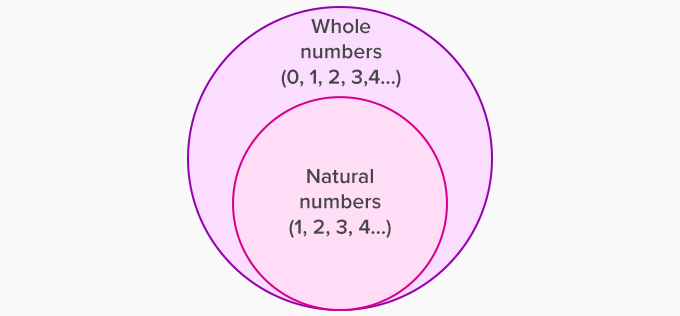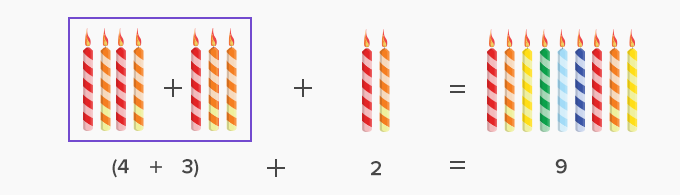Properties Of Addition - Definition with Examples

The Complete K-5 Math Learning Program Built for Your Child

• 30 Million Kids

Loved by kids and parent worldwide

• 50,000 Schools

Trusted by teachers across schools

• Comprehensive Curriculum

Aligned to Common Core

There are four properties of addition of whole numbers.

• Closure Property

• Commutative Property

• Associative Property

• Additive Identity Property

Before we start with the properties, let us revise what are whole numbers.

Whole numbers

Natural numbers (Counting numbers), including 0, form the set of whole numbersClosure Property:

The sum of the addition of two or more whole numbers is always a whole number.

Whole Number + Whole Number = Whole Number

For example, 2 + 4 = 6Here, both 2 and 4 whole numbers and their sum is 6, which also is a whole number.

Commutative Property

When we add two or more whole numbers, their sum is the same regardless of the order of the addends.

Example 1: 2 + 4 = 4 + 2 = 6The sum of both 2 + 4 and 4 + 2 is 6. That means, we can add whole numbers in any order.

Associative Property

When three or more numbers are added, the sum is the same regardless of the grouping of the addends.

For example (4 + 2) + 3 = (4 + 3) + 2Here, the addends are 2, 4 and 3. So, as per the associative property, the sum of the three numbers will remain the same, no matter how we group them.

So, (2 + 4) = 3 = (4 + 3) + 2 = 9